# 1.1 Moving Average (MA)

Moving Average (MA) is a commonly used means for analyzing time series. It can filter high-frequency noise and detect outliers. Based on different computation methods, common MA algorithms include simple moving average, weighted moving average, and exponential moving average. Assume that the MA time window is T and a time series is provided:

## 1.1.1 Simple Moving Average (SMA)

1. Smooth the noise data, that is, replacing the current time value with the moving average to filter noise.
2. Predict future values.

## 1.1.2 Weighted Moving Average (WMA)

As the Simple Moving Average (SMA) gives the same weight to all data points in the window, it is not sensitive to more recent data, and the predicted value may be lagging. Following this line of thinking, it will naturally give a higher weight to more recent data when computing the moving average, while giving a lower weight to older data within the window, so as to capture the recent changes faster. Thus, the Weighted Moving Average (WMA) and the Exponential Moving Average (EMA) are obtained.

## 1.1.3 Exponential Moving Average (EMA)

The Exponential Moving Average (EMA) is similar to the Weighted Moving Average (WMA), but the difference is that the weight of each value decreases exponentially instead of linearly. In addition, for the exponential decreases, no matter how old the data is, the coefficient of the data in this period will not decrease to 0, but only approach 0. Therefore, the EMA is actually an infinite series, that is, no matter how old the data is, it will play a certain role in computing the current EMA, but the weight of the data that is too far away from the current time is very low. In practical application, the EMA at time “t” can be obtained as follows:

# 1.2 Year-on-Year Ratio and Month-over-Month Ratio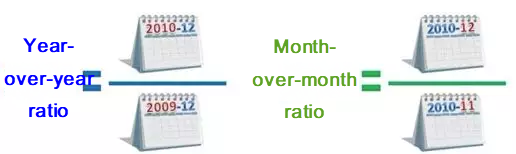Figure 2. Year-on-Year ratio and Month-over-Month ratio

# 1.3 Anomaly Detection of Time Series Metrics (STL + GESD)

STL is a single-dimension anomaly detection algorithm for time series metrics. The general idea is:

1. To decompose the metrics into STL time series first, to obtain the Seasonal, Trend, and Residual components, as shown in Figure 3.
2. To use the GESD (which stands for generalized extreme studentized deviate) algorithm to detect the anomaly of the Trend and Residual components.
3. To replace the mean and std statistics in the GESD algorithm with median and MAD (median absolute deviation), to improve the robustness against the outlier.
4. To output the anomaly score: abnorm_score = (value — median)/MAD, where the “value” is the current value, and “median” is the median of the sequence. A negative score indicates a decrease in anomalies, while a positive score indicates an increase in anomalies.

# 2.1 Single Feature, Which Conform to Gaussian Distribution

If the variable x follows the Gaussian distribution

# 2.2 Multiple Unrelated Features, All of Which Conform to Gaussian Distribution

Assume that an n-dimensional dataset looks like this:

# 2.3 Multiple Related Features, Which Conform to Multivariate Gaussian Distribution

Assume that an n-dimensional dataset is

# 2.4 Mahalanobis Distance

For a multi-dimensional column vector dataset D, assuming that

# 2.5 Boxplot

The boxplot algorithm does not require data to be subject to a specific distribution. For example, this method can be used even if the data distribution does not conform to Gaussian distribution. This method needs to compute the first quartile Q1 (25%) and the third quartile Q3 (75%). Let IQR = Q3-Q1, and then compute the outlier boundary points Q3 + λIQR and Q1- λIQR. Usually, λ takes a value of 1.5 (similar to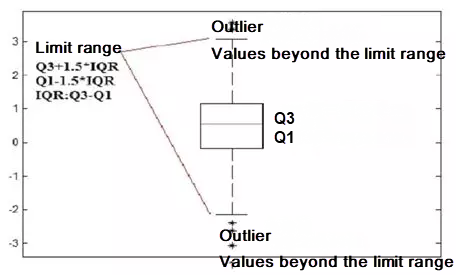Figure 4. Schematic diagram of boxplot algorithm

# 3.1 Angle-Based Outlier Detection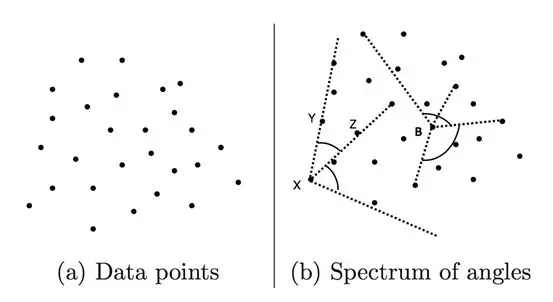Figure 5. Point set and angle

# 3.2 KNN-Based Outlier Detection

If D is a point set, then for any point

# 4. Linear Method (Matrix Decomposition and PCA Dimension Reduction)

The main idea of the matrix decomposition-based outlier detection method is to use Principal Component Analysis (PCA) to find outliers that violate the correlation between data. To locate these outliers, the PCA-based algorithm projects data from the original space to the principal component space, and then from the principal component space to the original space. For most data, if only the first principal component is used for projection and reconstruction, the error after reconstruction is small. However, for outliers, the error after reconstruction is relatively large. This is because the first principal component reflects the variance of normal points, and the last principal component reflects the variance of outliers.

# 5. Distribution

The policy is to compare the distribution of a feature of the benchmark traffic and that feature of the traffic to be detected.

# 5.1 Relative Entropy (KL Divergence)

The relative entropy (KL divergence) can measure the distance between two probability distributions. When two probability distributions are the same, their relative entropy is zero. If the difference between the two probability distributions increases, their relative entropy will increase accordingly. Therefore, the relative entropy can be used to compare the similarity of two probabilities. Given two probability distributions

# 5.2 Chi-square Test

A chi-square test can be used to compare the difference between the expected result and the observed result by using the test statistics

# 7.1 Maximal Connected Subgraph

In the undirected graph G, vertex A is connected to vertex B if a path exists between them. Graph G contains several subgraphs, where each vertex is connected to every other vertex in the same subgraph but is separated from those in other subgraphs. These subgraphs of graph G are maximum connected subgraphs.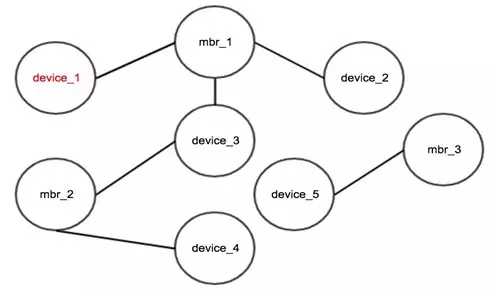Figure 9. Maximal connected subgraph result

# 7.2 Label Propagation for Clustering

The label propagation algorithm for graph clustering is used to divide a graph into subgraphs based on the topology of the graph so that the links between the nodes in a subgraph are more than the links between the subgraphs. The basic idea of the label propagation algorithm is that the label of a node depends on the label information of its neighboring nodes. The impact level is decided by the node similarity. Labels are propagated, iterated and updated to reach a stable status. Two subgraphs are obtained for the nodes in Figure 10 after the labels are propagated for clustering, where nodes 1, node 2, node 3, and node 4 belong to the same subgraph and node 5, node 6, node 7, and node 8 belongs to the other subgraph.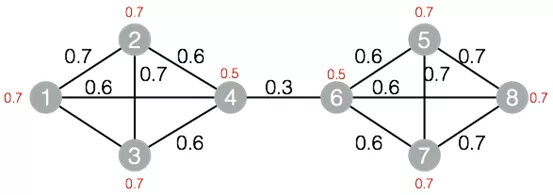Figure 10. Graph structure of the label propagation algorithm for clustering

# 8. Behavior Sequence (Markov Chain)

As shown in Figure 11, users have five behavior states on the search engine: page request (P), search (S), natural search result (W), add click (O), and page turning (N). State transition may happen between states. A chain composed of several behavior states can be considered a Markov chain.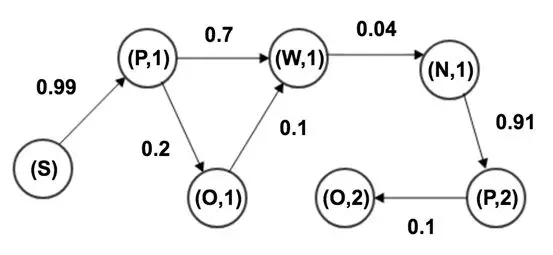Figure 11. User behavior state chart

# 9. Supervised Models

The preceding methods are relatively simple and easy to implement, because none of them involve supervision. However, because some of these methods only use a small number of features each time, we need to maintain lots of policies to intercept fraud in a comprehensive way. In addition, the effect of combining some of the aforementioned methods for multiple features depends on personal experience. Supervised models can automatically combine many features and provide a more powerful generalization ability.

# 9.1 Machine Learning Model GBDT

Sample: Use the fraud samples mined by using the preceding unsupervised methods as the training samples. If the number of the fraud samples is still small, use Synthetic Minority Over-sampling Technique (SMOTE) or Generative Adversarial Net (GAN) to generate more fraud samples. Then train a gradient boosting decision tree (GBDT) and use the transformation data to evaluate the model.

# 9.2 Deep Learning Model Wide & Deep

Wide & Deep extracts wide features and deep features respectively and then merge them for training. The model structure is shown in Figure 12. Wide refers to the LR of high-dimensional features and feature combinations. LR is efficient, scalable and strongly interpretable. The constant enhancement of feature combinations will facilitate memorization of the model judgement. However, the generalization ability of LR is weak.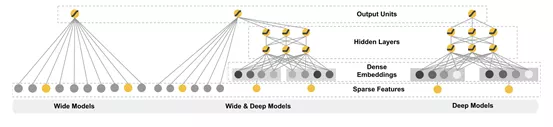Figure 12. Wide & Deep model

# 10.1 Common Threshold Selection Methods

All the preceding methods involve the computation of anomaly threshold. We can select a threshold by using the following methods and then use the transformation data to verify the rationality of the selected threshold.

1. Unsupervised methods: Use the quantile threshold and locate the inflection point of the distribution curve of historical data.
2. Supervised models: Check the accuracy curve and the recall curve of the validation sets.

# 10.2 Transform Non-Gaussian Distributions to Gaussian Distributions

Some features do not conform to the Gaussian distribution. We can use functions to transform them so that they become compliant with the Gaussian distribution and the aforementioned statistics methods can be used. A common transformation function is , where c is a non-negative constant. Another common transformation function is , where c is a fraction between 0 and 1.

# References:

 Charu C, Aggarwal, et al. Outlier Analysis Second Edition, Springer. 2016
 Varun Chandola, Arindam Banerjee, et al. Anomaly Detection: A survey, ACM Computing Surveys. 2009
 Kalyan Veeramachaneni, Ignacio Arnaldo, et al. AI2: Training a big data machine to defend, In Proc. HPSC and IDS. 2016
 Liu, Fei Tony, Kai Ming Ting, and Zhi-Hua Zhou, et al. Isolationforest, ICDM. 2008
 Cheng H T, Koc L, Harmsen J, et al. Wide & Deep Learning for Recommender Systems, ACM Computing Surveys. 2016
 SMOTE: Synthetic Minority Over-sampling Technique, JAIR. 2002

# Original Source

--

--## Alibaba Cloud

Follow me to keep abreast with the latest technology news, industry insights, and developer trends. Alibaba Cloud website:https://www.alibabacloud.com